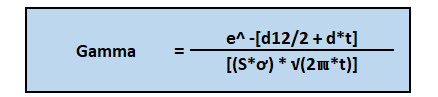# Gamma

• Updated on

Gamma is a Greek alphabet used in the investing world to reflect the change in the rate of an options price about an underlying asset. It is a measurement tool for price changes explicitly used for options. It is the second derivative of an options price, the first being its’ Delta.  A positive Gamma is an indication that an option traders’ trading position is also favourable. Usually, the Gamma value change is more significant than the Delta value change resulting from an upward or downward movement in an underlying assets’ price. While Delta is the speed of change in the price of an asset concerning its underlying’s price, Gamma talks about the acceleration, i.e., the rate of change in price movement. In other words, Gamma measures movement (upward/ downward) risk. The gamma value, which is related to whether the option is long or short in the market, likewise ranges from 0 to 1. A long position on a call or put option gives a positive gamma. On the other hand, a short position on a call or put option gives negative Gamma.

Highlights
• Gamma reflects the change in the rate of an options price concerning an underlying asset. It talks about acceleration, i.e., the rate of change in price movement.
• Gamma value ranges between 0 and 1 and is linked to whether the option is long or short in the market.
• It is a valuable metric to measure risk and is often used by traders to study the risk associated with underlying price movement.

## How is gamma calculated?Source: © Brandelmeier | Megapixl.com

Often organisations use either spreadsheets or software for computing Gamma, as it is a bit complex. But its manual computation considers the following values- asset dividend yield in case of dividend-paying stocks, spot and strike price of the underlying stock, its’ standard deviation, the option’s time to expiry, the risk-free rate of return is also considered.

Gamma value is derived using the following steps:

• Firstly, the spot price of the underlying asset is taken then, the strike price of the underlying asset is taken from the details of the option.
• Next, we can check whether any dividend is paid on the stock.
• After this, the maturity of the option or time left till expiration is also considered.
• Then the standard deviation of the underlying asset is measured, and the risk-free rate is taken, usually the return of government bonds.
• Finally, all these values are put in the formula to derive the Gamma value.

The formula of Gamma-## What is the importance of gamma?

• Traders can use gamma to correct issues related to convexity involved in hedging strategies.
• Portfolios of considerable value require additional precision when engaging in hedging.
• Gamma value is used to maintaining the gamma- hedging of a portfolio.
• Gamma positions, use a risk graph to know when a trader is in danger of losing too much money.
• Gamma can help one estimate the price and reduce risks and gain profits.

What impacts the value of gamma?

The value of gamma is dynamic and keeps changing over time. Two key factors impact the value of the gamma over some time, viz. time to expiry and the volatility of the underlying assets price. The gamma curve is usually flat in case of high volatility and shows very sharp movements in case of low volatility. When the volatility is high, a reasonable degree of time value is already priced into the options. It limits the scope for changes in Delta, making the gamma curve much flatter in times of high volatility. Thus, gamma is affected by these two factors only.

What is gamma squeeze?